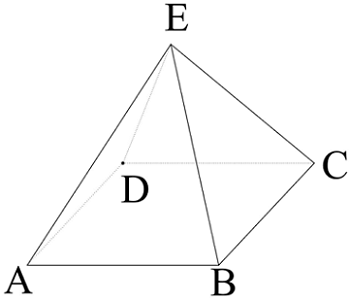# Resistor PyramidJo has 8 identical resistors with resistance $r$. He decides to build a Resistor Pyramid for his Physics project. Being a curious boy, he tries to find out the equivalent resistance between $A$ and $B$ using his ohmmeter. Find the equivalent resistance that the ohmmeter shows up when $r = 1 \Omega$.

If the resistance can be expressed in the form of $\dfrac{\alpha}{\beta}$, where $\alpha$ and $\beta$ care coprime positive integers, submit your answer as $\alpha + \beta$.

×